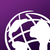# Counting a specific responses to different questions

139
2
07-08-2022 01:52 PMNew Contributor III

Connect v3.14.274

Hello! I have a survey with multiple select_one questions that have yes/no answers. How would I go about creating a running tally that displays at the bottom of the survey, in real time, how many times "Yes" has been selected?

Thank you!

2 Repliesby
Occasional Contributor III

Create one calculate for every select_one.Then a final calculate to add all of those together. This will give you a running tally of questions answered "yes".by
Occasional Contributor III

I think the message I was responding to was deleted. I'll add this here in case anyone else was curious about how to tally up those calculate fields.

Simply add all of the calculates together in one final calculate similar to this:

``\${question1_calc}+\${question2_calc}+\${question3_calc}+...+\${question100_calc}``

You can add as many items as you need.

If set-up like my example above, each of the calculates will have an integer value of either 1 or 0.

In the end, S123 will only see (for example):

1+0+0+1+0+1 and will give you a total of 3